# How to show percentages in Excel

In this short tutorial, you will find many helpful details about Excel percent format and learn how to format existing values as per cents, how to show percentage in empty cell and change numbers to percentages as you type.

In Microsoft Excel, displaying values as percentages is very straightforward. To apply the percent format to a given cell or several cells, select them all, and then click the Percent Style button in the Number group on the Home tab: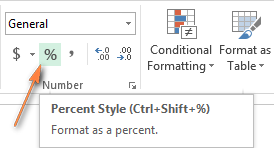Even a faster way is pressing the Ctrl + Shift + % shortcut (Excel will remind you of it every time you hover over the Percent Style button).

Although formatting numbers as percentages in Excel takes just a single mouse click, the results may vary depending on whether you apply the percent formatting to existing numbers or empty cells.

## Formatting existing values as percentage

When you apply the Percentage format to cells that already contain numbers, Excel multiplies those numbers by 100 and adds the per cent sign (%) at the end. From Excel's viewpoint, this is the correct approach since 1% is one part of one hundred.

However, this way does not always work right. For example, if you have 20 in cell A1 and you apply the percentage format to it, you will get 2000% as the result, and not 20% as you might expect.

Possible workarounds:

• Calculate numbers as percentages before applying the percent format. For example, if your original numbers are in column A, you can enter the formula `=A2/100` in cell B2 and then copy it down to all other cells in column B. Then select the entire column B and click the Percent Style. You will get a result similar to this: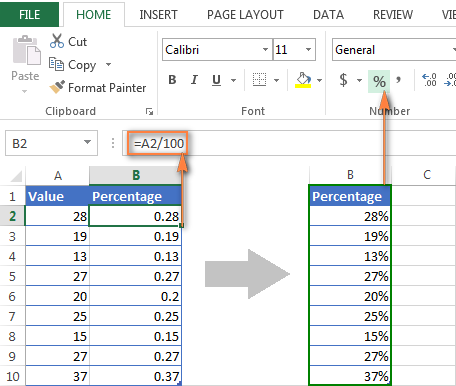Finally, you can replace the formulas with values in column B, copy them back to column A and delete column B if you do not need it any longer.

• If you want to apply the percentage formatting to just a few numbers, you can type a number in its decimal form directly into the cell. For example, to have 28% in cell A2, type 0.28 and then apply the percentage format.

## Applying percentage format to empty cells

Microsoft Excel behaves differently when you enter numbers into empty cells pre-formatted as Percentage:

• Any number equal to or greater than 1 is converted to a percent by default. For example, 2 is turned into 2%, 20 into 20%, 2.1. into 2.1% and so on.
• Numbers smaller than 1 without a preceding zero are multiplied by 100. For example, if you type .2 in a percentage preformatted cell, you'll see 20% in that cell. However, if you enter 0.2 in the same cell, 0.2% will appear exactly as it should.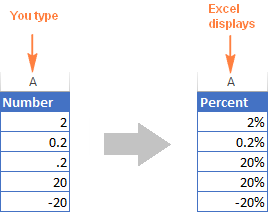## Display numbers as percentages as you type

If you type 20% (with the percentage sign) directly in a cell, Excel will understand that you are entering a percent and will apply percentage formatting automatically.

#### Important note!

When applying the percentage formatting it Excel, please keep in mind that it is nothing more but a visual representation of a real value stored in a cell. The underlying value is always stored in decimal form.

In other words, 20% is stored as 0.2, 2% is stored as 0.02, 0.2% is 0.002, etc. When performing calculations, Excel always deals with the underling decimal values. Please remember this fact when referencing percent cells in your formulas.

To see the real value behind percentage formatting, right-click the cell, click Format Cells (or press Ctrl + 1) and look in the Sample box under the General category on the Number tab.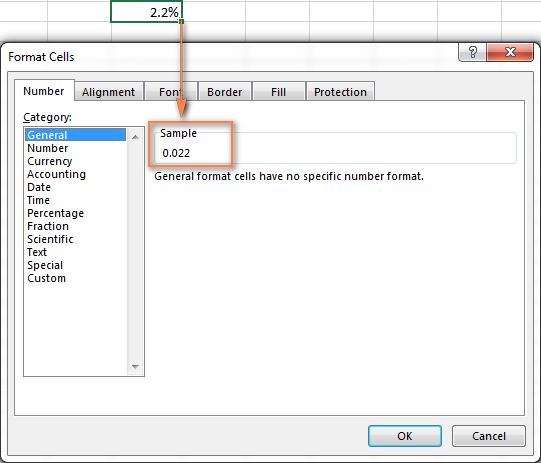## Tips to display percentages in Excel

Showing percentage in Excel seems to be one of the earliest tasks, right? But experienced Excel users know that a path to the goal almost never runs smooth :)

#### 1. Display as many decimal places as you want.

When applying percentage formatting to numbers, Excel 2016, 2013 and 2010 display their rounded versions with no decimal places, which may cause some confusion. For example, apply the percent format to an empty cell and then type 0.2 into it. What do you see? In my Excel 2013, I see 0% though I know with certainty it should be 0.2%.

To see the actual percentage instead of a rounded version, you just need to increase the number of decimal places showing. To do this, open the Format Cells dialog either by pressing Ctrl + 1 or right-clicking the cell and selecting Format Cells… from the context menu. Make sure the Percentage category is selected and specify the desired number of decimal places in the Decimal places box.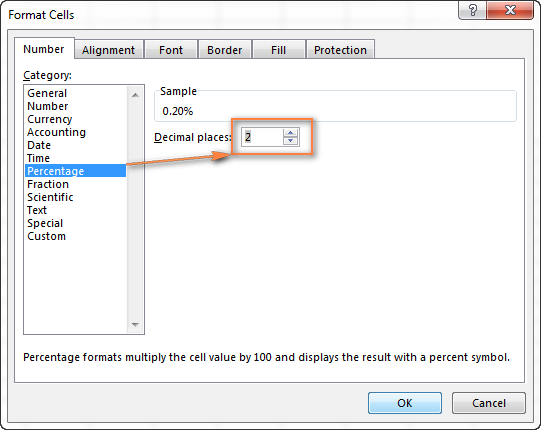When done, click the OK button to save your settings.

Alternatively, you can control the number of displayed decimal places, by clicking the Increase Decimal or Decrease Decimal icons on the ribbon (Home tab > Number group):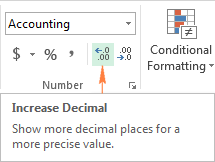#### 2. Apply a custom format to negative percentages.

If you want to format negative percentages in a different way, say in red font, you can create a custom number format. Open the Format Cells dialog again, navigate to the Number tab > Custom category and enter one of the below formats in the Type box:

• 00%;[Red]-0.00% - format negative percentages in red and display 2 decimal places.
• 0%;[Red]-0% - format negative percentages in red without any decimal places showing.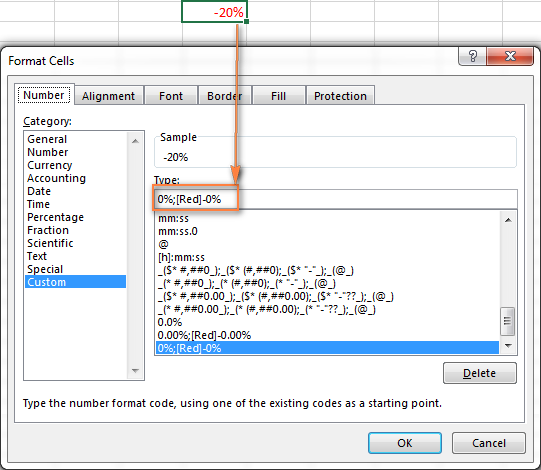You can find more details about this formatting technique in the Display numbers as percentages article by Microsoft.

#### 3. Format negative percentages using Excel conditional formatting.

Compared to the previous method, Excel conditional formatting is more versatile and it lets you display negative percentages, e.g. percent decrease, in any format of your choosing.

The fastest way to create a conditional formatting rule for negative percentages is to click Conditional formatting > Highlight cells rules > Less than and put 0 in the "Format cells that are LESS THAN" box: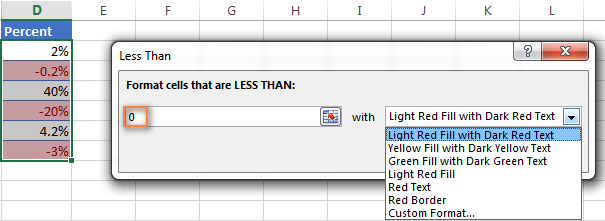Then you choose one of the formatting options from the drop-down list on the right, or click Custom Format... at the end of the list to set up for own formatting.

This is how you work with Excel percent format. Hopefully, this knowledge will help you avoid a lot of headache when you in the future. In the next articles, we are going to learn how to calculate percentages in Excel and write formulas for percent change, percentage of a total, compound interest and more. Please stay tuned and thank you for reading!

### 28 Responses to "How to show percentages in Excel"

1.Gurpreet says:

How can I calculate the prcentage of \$8660 in one cells its a revenue

•Svetlana Cheusheva (Ablebits.com Team) says:

Hi Gurpreet,

Simply multiply the original number by the desired percentage. For example:
=A1*10% or =A1*0.1

•suresh says:

1
1
4
10
6
19
25
23

Total value
89. how can i find percentage of all these values

2.Jaspal Singh Bagnal says:

Hi,
Is there a way I can use any function to convert a part of the calculation to percentage?
To give an example - I'm using a formula to show notes in my report where I want to show the max savings (in %) from a particular location/country (dynamic).
A sample formula would be -
="Maximum savings from "&ROUND(MAX(H23:K23),2)&" comes by transitioning to "&(HLOOKUP(MAX(H23:K23),H23:K24,2,FALSE))

In the above example:
1. "ROUND(MAX(H23:K23),2)" is used to calculate and show the percentage (somehow it is showing in decimals currently). This is what I want to show as %.
2. "(HLOOKUP(MAX(H23:K23),H23:K24,2,FALSE))" is used to calculate and show the location where the maximum savings are coming from.

The end result as seen now is "Maximum Savings of 0.8 comes by transition to India". :(
Suggestions would be really appreciated. thanks in advance.

3.Mayank Yadav says:

Thanks

4.ANEEZ says:

have an unusual problem with Excel 2010. I have a file created with Excel 2013 with percentages and money values and it all looks fine in 2013. When I open it in 2010 it does not show the percentage, but shows me 0.9540 instead of 95.40%. PLZZZZ HELP ME

5.Jeff says:

If I have a line item that has a \$0 budget but spent \$400. How do I show that in percent?

6.Santhosh says:

2000/- and 200/- less amount
What is the Percentage of Discount

7.MARIA PONDES says:

Help please. How do I calculate the discount percentage of a product. EG item is \$1000 and now discounted to Sales Price of \$500 What formula do I use to show 50%
Thank you

8.asim says:

When i add percentage sign ..... with 20 it becomes 2000% ..... i need just only 20 .00%

•Svetlana Cheusheva (Ablebits.com Team) says:

Hi Asim,

You can type %20 in an empty cell and Excel will display it properly.
However, to format existing numbers as percentages, you have to divide them by 100 first, and then apply the Percent format. For the detailed explanation, please see Format existing values as percentage

9.Kim says:

What is the formula for converting a raw mark that is not out of 100 (e.g 46/60) into a percentage? Thank you

•Gennady Terekhov (Ablebits.com Team) says:

Hello,

=46/60*100

Or

=TEXT(46/60,"0.00%")

•Sufiyana says:

Thank you for the simple formlula...it really helped me

10.Neha Malik says:

I have one question.
Suppose you entered a value as 0.72 in a number field having percentage format. But in place of 72% , it is displaying 100%. Can you find out a reason.

11.suresh says:

i want find percentage of few values

books 20
paper 30
table 100
chairs 20

total 170
how can i find the percentage.

12.muradali says:

1099
494

13.Hassan Jatta says:

Let me thank you very much for the excel knowledge you are sharing. Please keep it up and know that we are very grateful.

14.someone says:

I have values in my excel in columns as
48GB 78GB 21GB
3.9TB 5.3TB 9.2TB

where GB is Gigabytes and TB is terrabytes I have to calculate percentages of above data but due to GB and TB in numbers I am getting errors. How can this be sorted out.

•Doug says:

I think there are at least two ways to solve your problem.
If you don't have many of these rows to split, just use the Text-to-Columns tool in Excel. Under Data choose the Text-to-Columns use the fixed width and that should do it. What I did when I was practicing with your sample data was to copy and Paste Special/Transpose it so I could get it into columns so I could split the top three into columns and then the second three.
If you have a lot of this data you can use this formula. It will split out the numbers for you. It needs to be entered with CTRL+Shift+Enter because it is an array. You can change E9 to the address you need.

=LOOKUP(10^99,--MID("|"&E9,SMALL(IF(((--ISNUMBER(--MID("|"&E9, ROW(\$1:\$1003),1))=0)*ISNUMBER(--MID("|"&E9,ROW(\$2:\$1004),1))),ROW(\$2:\$1004)),1), ROW(\$1:\$1003)))

15.Rob says:

Trying to learn how to use excel better at work I need to try and find the percentage of staff who have completed training so let's say 30 staff and in the B column are all the dates I.e. 23/09/2017 how do I work out the percentage of the number of staff with dates next to there names. Then could I put the total percentage onto a different page. Can anyone help

16.Michael McEveley says:

I am trying to deduct a percentage (%) from a cell into another cel?

A B C D E F G H I J
1. Model Outlet 2019 LIST -25% -30% -33% -35% -37% -40%
2.LB-480 2" 575.00
3.

For example i want to have E3 to show D9-25%

17.gautham says:

F2 value 69050 how to calcutae 20%

Price AMC
69050 calculate for this AMC
69050
69050
69050

18.Audrey Day says:

If I am trying to calculate room occupancy with time how to do it. for example,

There are a total of 40 hours the class can be used per week from 8-4.
Classes are
8:00 am to 10:40 am 2 classes per week at 5.20 hours
9:30 am to 10:45 am 2 classes per week at 2.30 hours

How do I find the percentage of time used of the classroom. If there are 40 total hours the class can be used and one class is at 5.20 hours of usage per week and another is 2.30 hours per week - what is the percentage of use time for each individual class out of 40 hours.

Thanks,
Audrey

19.Shobin says:

How can I find the 20 % mark of a students mark, I want to prepare progress report. I just want to know the formula.

20.Katherine says:

I have a a document showing whether someone was late, early or on time. I need to get the percentage of how any times someone was late, early or on time. The thing is, is that it's marked down as "X" in the appropriate column and I don't know how to get the percentage. Help, please?!

21.SAGAR says:

total class 4
one student present in class 4
how to use presentage ratio 100
send me presentage formula

22.ALI RAZA says: